Updating search results...

# 37 Results

View
Selected filters:
• MI.Math.Content.5.NF.A.1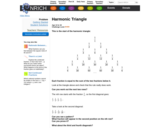Unrestricted Use
CC BY
Rating
0.0 stars

This Nrich problem provides a fraction-based challenge for students who already possess a good understanding of fraction addition and subtraction, and it leads to algebraic manipulation of that same process.

Subject:
Mathematics
Material Type:
Activity/Lab
06/17/2021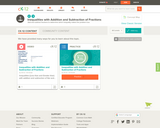Conditional Remix & Share Permitted
CC BY-NC-SA
Rating
0.0 stars

This short video and interactive assessment activity is designed to teach fifth graders about inequalities with addition and subtraction of fractions.

Subject:
Mathematics
Numbers and Operations
Material Type:
Assessment
Interactive
Lecture
Provider:
CK-12 Foundation
Provider Set:
CK-12 Elementary Math
04/03/2018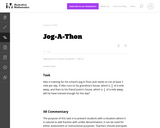Unrestricted Use
CC BY
Rating
0.0 stars

The purpose of this task is to present students with a situation where it is natural to add fraction with unlike denominators; it can be used for either assessment or instructional purposes.

Subject:
Mathematics
Numbers and Operations
Material Type:
Activity/Lab
Provider:
Illustrative Mathematics
Provider Set:
Illustrative Mathematics
Author:
Illustrative Mathematics
08/07/2012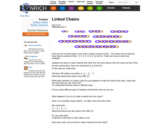Unrestricted Use
CC BY
Rating
0.0 stars

This Nrich activity is an unusual context in which pupils can consolidate recognizing, finding, naming and writing fractions. The rich environment also gives them the opportunity to identify, name and write equivalent fractions of a given fraction, represented visually as a chain. Furthermore, learners will be adding and subtracting fractions with the same denominators and denominators which are multiples of the same number.

Subject:
Mathematics
Material Type:
Activity/Lab
06/18/2021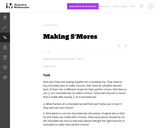Unrestricted Use
CC BY
Rating
0.0 stars

The purpose of this instructional task is to motivate a discussion about adding fractions and the meaning of the common denominator. The different parts of the task have students moving back and forth between the abstract representation of the fractions and the meaning of the fractions in the context.

Subject:
Mathematics
Numbers and Operations
Material Type:
Activity/Lab
Provider:
Illustrative Mathematics
Provider Set:
Illustrative Mathematics
Author:
Illustrative Mathematics
08/10/2012Conditional Remix & Share Permitted
CC BY-NC-SA
Rating
0.0 stars

In this activity, students will focus on subtracting fractions, starting with single-digit denominators and working up.

Subject:
Mathematics
Ratios and Proportions
Material Type:
Activity/Lab
Game
Interactive
08/04/2020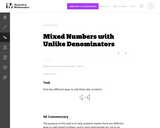Unrestricted Use
CC BY
Rating
0.0 stars

The purpose of this task is to help students realize there are different ways to add mixed numbers and is most appropriate for use in an instructional setting.

Subject:
Mathematics
Numbers and Operations
Material Type:
Activity/Lab
Provider:
Illustrative Mathematics
Provider Set:
Illustrative Mathematics
Author:
Illustrative Mathematics
08/08/2012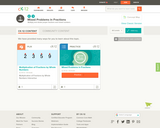Conditional Remix & Share Permitted
CC BY-NC-SA
Rating
0.0 stars

This short video and interactive assessment activity is designed to teach fifth graders about mixed problems in fractions.

Subject:
Mathematics
Numbers and Operations
Material Type:
Assessment
Interactive
Lecture
Provider:
CK-12 Foundation
Provider Set:
CK-12 Elementary Math
04/03/2018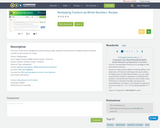Conditional Remix & Share Permitted
CC BY-NC-SA
Rating
0.0 stars

In this lesson designed to enhance literacy skills, students examine how to multiply fractions by whole numbers in the context of a recipe.

Subject:
Mathematics
Material Type:
Activity/Lab
Author:
WGBH Educational Foundation
Walmart Foundation
08/05/2020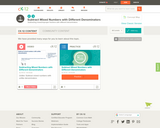Conditional Remix & Share Permitted
CC BY-NC-SA
Rating
0.0 stars

This short video and interactive assessment activity is designed to teach fifth graders about subtracting mixed numbers with different denominators.

Subject:
Mathematics
Numbers and Operations
Material Type:
Assessment
Interactive
Lecture
Provider:
CK-12 Foundation
Provider Set:
CK-12 Elementary Math
04/03/2018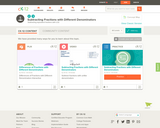Conditional Remix & Share Permitted
CC BY-NC-SA
Rating
0.0 stars

This short video and interactive assessment activity is designed to teach fifth graders about subtracting fractions with different denominators.

Subject:
Mathematics
Numbers and Operations
Material Type:
Assessment
Interactive
Lecture
Provider:
CK-12 Foundation
Provider Set:
CK-12 Elementary Math
04/03/2018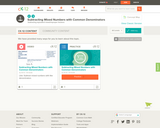Conditional Remix & Share Permitted
CC BY-NC-SA
Rating
0.0 stars

This short video and interactive assessment activity is designed to teach fifth graders about subtracting mixed numbers with common denominators.

Subject:
Mathematics
Numbers and Operations
Material Type:
Assessment
Interactive
Lecture
Provider:
CK-12 Foundation
Provider Set:
CK-12 Elementary Math
04/03/2018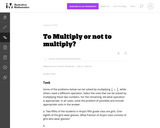Unrestricted Use
CC BY
Rating
0.0 stars

This series of word problems requires students to determine which problems can be solved by multiplying 1/8_2/5.

Subject:
Mathematics
Numbers and Operations
Material Type:
Activity/Lab
Provider:
Illustrative Mathematics
Provider Set:
Illustrative Mathematics
Author:
Illustrative Mathematics
08/21/2012Conditional Remix & Share Permitted
CC BY-NC-SA
Rating
0.0 stars

UMCDC 5th Grade Engage NY Math Module 3 Topic B (20-21) In Topic B, students use the familiar rectangular fraction model to add and subtract fractions with unlike denominators. Students make like units for all addends or both minuend and subtrahend. First, they draw a wide rectangle and partition it with vertical lines as they would a tape diagram, representing the first fraction with a bracket and shading. They then partition a second congruent rectangle with horizontal lines to show the second fraction. Next, they partition both rectangles with matching lines to create like units.

Subject:
Mathematics
Material Type:
Homework/Assignment
Author:
UMCDC
06/11/2021Conditional Remix & Share Permitted
CC BY-NC-SA
Rating
0.0 stars

UMCDC 5th Grade Engage NY Math Module 3 Topic C (20-21) In Topic C, students use the number line when adding and subtracting fractions greater than or equal to 1. The number line helps students see that fractions are analogous to whole numbers. The number line makes it clear that numbers on the left are smaller than numbers on the right, which leads to an understanding of integers in Grade 6. Using this tool, students recognize and manipulate fractions in relation to larger whole numbers and to each other. For example, “Between which two whole numbers does the sum of 1 2 3 and 5 3 4 lie?”

Subject:
Mathematics
Material Type:
Homework/Assignment
Author:
UMCDC
06/11/2021Conditional Remix & Share Permitted
CC BY-NC-SA
Rating
0.0 stars

UMCDC 5th Grade Engage NY Math Module 3 Topic D (20-21) Topic D opens with students estimating the value of expressions involving sums and differences with fractions. “Will your sum be less than or greater than one half? One? How do you know?” Though these conversations have been embedded within almost every Student Debrief up to this point, by setting aside an instructional day to dig deeply into logical arguments, students see that it is very easy to forget to make sense of numbers when calculating. This is really the theme of this topic—reasoning while using fractions.

Subject:
Mathematics
Material Type:
Assessment
Homework/Assignment
Author:
UMCDC
06/11/2021Conditional Remix & Share Permitted
CC BY-NC-SA
Rating
0.0 stars

UMCDC 5th Grade Engage NY Math Module 3 (20-21) In Module 3, students’ understanding of addition and subtraction of fractions extends from earlier work with fraction equivalence and decimals. This module marks a significant shift away from the elementary grades’ centrality of base ten units to the study and use of the full set of fractional units from Grade 5 forward, especially as applied to algebra.

Subject:
Mathematics
Material Type:
Assessment
Homework/Assignment
Author:
UMCDC